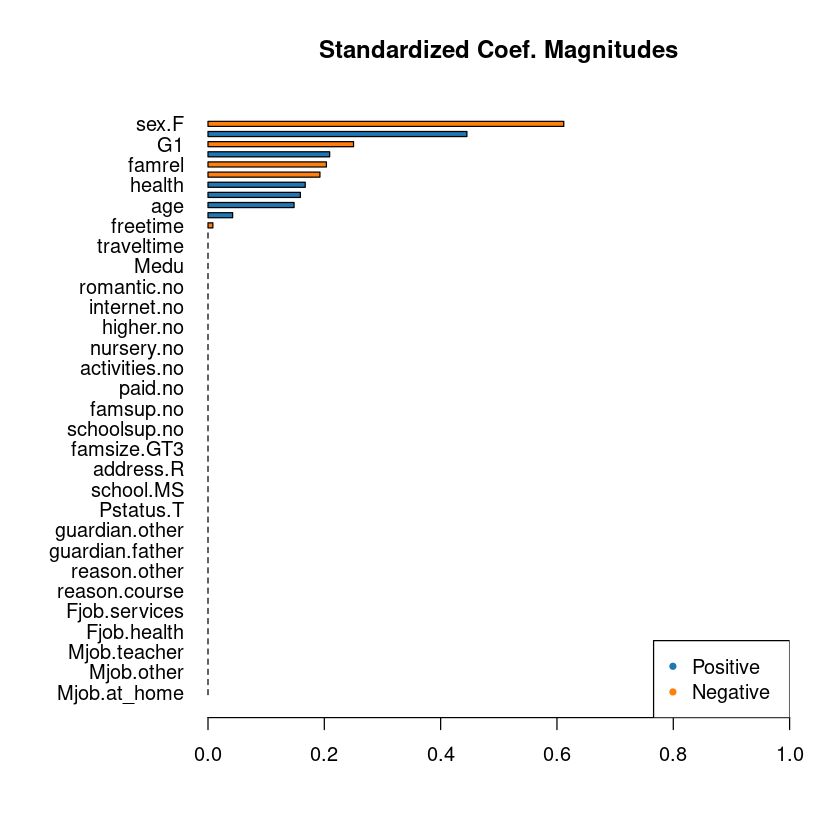# Machine Learning Linear Regression And Regularlization

Linear regression is a model to predict a variable based on independent variables. The model assumes linear relationship between dependent and independent variables. Below represents a simple linear regression equation.

y = a + c1x1 + c2x2

In above equation y is a dependent variable and x1,x2 are independent variables. a is a intercept, c1 and c2 are coefficients. In above equation, we are trying to predict y based on x1 and x2 variables.

In this post, I will do an example of linear regression and regularization using Maching Learning package H2o. H2o is a great library and offers lot of techniques right out of the box.

I will use students alcohol data which I downloaded from following UCI website...

archive.ics.uci.edu/ml/datasets/student+performance

Before we delve in to our data analysis, Make sure you have following installed and working...

## Required

R installed
Anaconda 3.7 installed
H2o installed - Check out how to install R and H2o

In your R repl, lets import the H2o package.

In :
library(h2o)
h2o.init()


Lets import our data file student-mat.csv

In :
st_mat <- h2o.importFile('student-mat.csv')

  |======================================================================| 100%


Lets look at first two rows using head method.

In :
head(st_mat,2)

A data.frame: 2 × 33
<fct><fct><dbl><fct><fct><fct><dbl><dbl><fct><fct><dbl><dbl><dbl><dbl><dbl><dbl><dbl><dbl><dbl><dbl>
1GPF18UGT3A44at_hometeacher4341136566
2GPF17UGT3T11at_homeother 5331134556

Lets look at the column names also.

In :
colnames(st_mat)

1. 'school'
2. 'sex'
3. 'age'
5. 'famsize'
6. 'Pstatus'
7. 'Medu'
8. 'Fedu'
9. 'Mjob'
10. 'Fjob'
11. 'reason'
12. 'guardian'
13. 'traveltime'
14. 'studytime'
15. 'failures'
16. 'schoolsup'
17. 'famsup'
18. 'paid'
19. 'activities'
20. 'nursery'
21. 'higher'
22. 'internet'
23. 'romantic'
24. 'famrel'
25. 'freetime'
26. 'goout'
27. 'Dalc'
28. 'Walc'
29. 'health'
30. 'absences'
31. 'G1'
32. 'G2'
33. 'G3'

To check number of rows, we can do using h2o.nrow.

In :
h2o.nrow(st_mat)

395

For linear regression, we should check how many columns are there. We can do with command h2o.ncol.

In :
h2o.ncol(st_mat)

33

One of most important thing about linear regression is chosing the right set of independent variables for our dependent variable.

For our dependent variable which is the variable we want to predict, Lets us pick "Walc" which is column number 28.

Walc - weekend alcohol consumption (numeric: from 1 - very low to 5 - very high)

Basically we are trying to predict weekend alcohol consumption. Lets see which of the variables help us doing that.

To train our Linear regression model, let us split our data in the ratio of 80% to 20% using h2o.splitFrame.

In :
students.splits <- h2o.splitFrame(data =  st_mat, ratios = .8)

In :
train <- students.splits[]
valid <- students.splits[]


Ok now we got our train and validation set separated.

In :
y = 28


Lets take out Walc and Dalc (daily alcohol consumption) from our independent variables.

In :
x=-match(c("Walc","Dalc"),names(st_mat))


Ok now let us run our linear regression model. For that we can use h2o.glm package. glm stands for generalized linear regression models.

## H2o Generalized Linear Regression Model (GLM)

In :
students.glm <- h2o.glm(x=x,y=y, training_frame = train,
validation_frame = valid,remove_collinear_columns = TRUE)

  |======================================================================| 100%


Ok since it is a small data set, the model just ran instantly.

Now we can print out the glm model coefficients using h2o.std_coef_plot

In :
h2o.std_coef_plot(students.glm)From the above graph we can look at the positive and negative parameters. Lets print the model coefficients to actually know their magnitudes.

Lets check which parameters are affecting positively to alcohol consumption.

We can use model$coefficients to access the coefficients of the variables of our linear regression. In : coeff_vector = students.glm@model$coefficients
print(coeff_vector[coeff_vector > 0])

 Intercept        age   failures      goout     health   absences         G2
0.43908352 0.11540452 0.05622664 0.40241119 0.12427294 0.01856066 0.05650706


As we see above, other than intercept , age , failures, goout, health, absences, G2 (second period Grade) all affect positively.

Lets see if any parameters which affect the alcohol consumption negatively.

In :
print(coeff_vector[coeff_vector < 0])

       sex.F    studytime       famrel     freetime           G1
-0.611686028 -0.225279062 -0.228980650 -0.008235832 -0.074973142


Female, studetime, famrel(quality of family relatives), freetime and (first period grade) all affect the weakly alcohol consumption negatively.

If we do model$model_summary, we can see which model type has been run by h2o default. In : students.glm@model$model_summary

A H2OTable: 1 × 7